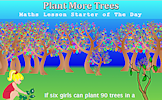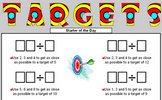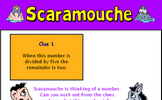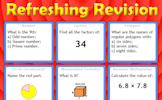# Ratio and fractions

### Term 2 starting in week 7 :: Estimated time: 2 weeks

• Compare quantities using a ratio (review)
• Link ratios and fractions (review)
• Share in a ratio (given total or one part) (review)
• Use ratios and fractions to make comparisons
• Link ratios and graphs (review)
• Solve problems with currency conversion
• Link ratios and scales (review)
• Use and interpret ratios of the form $$1 : n$$ and $$n : 1$$
• Combine a set of ratios
• Mixed ratio problems

For higher-attaining pupils:

• Ratio in area problems
• Ratio in volume problems

This page should remember your ticks from one visit to the next for a period of time. It does this by using Local Storage so the information is saved only on the computer you are working on right now.

## Lesson Starters

Here are some suggestions for whole-class, projectable resources which can be used at the beginnings of each lesson in this block.

### 1st Lesson#### Aunty Dotty

A real life situation requiring the division an amount of money according to a given ratio.

### 2nd Lesson#### Plant More Trees

If six girls can plant 90 trees in a day. How many trees can ten girls plant in a day? The unitary method.

### 3rd Lesson#### Word Search

Create your own mathematical word search for the whole class to enjoy.

### 4th Lesson#### Targets

Use the given numbers to produce a calculation to get as close as possible to the given target.

### 5th Lesson#### Scaramouche

Can you work out from the five clues given what the mystery number is?

### 6th Lesson#### Refreshing Revision

It is called Refreshing Revision because every time you refresh the page you get different revision questions.

Some of the Starters above are to reinforce concepts learnt, others are to introduce new ideas while others are on unrelated topics designed for retrieval practice or and opportunity to develop problem-solving skills.

White Rose ResourcesEnd of block assessments provide a quick progress check at the end of each block of learning to make sure students have understood the content covered. This Scheme of Learning was produced by White Rose Maths and is used here with permission granted on 30th June 2021.For All: